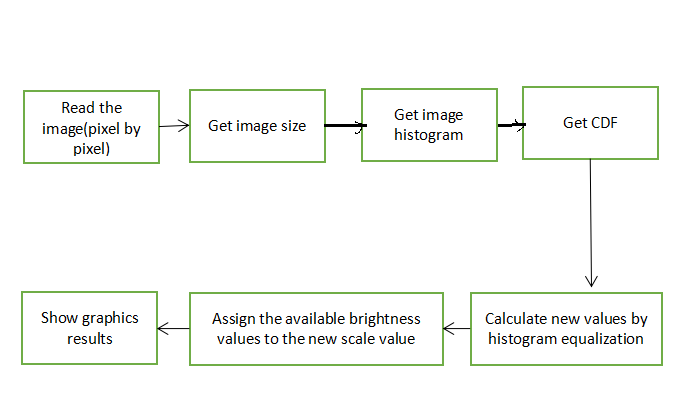How to Perform Contrast Enhancement Using Histogram Equalization in MATLAB?

• Difficulty Level : Expert
• Last Updated : 22 Nov, 2021

Image enhancement is a manner to enhance the appearance of photographs/images to human viewers or to image processing device performance. Image Enhancement strategies may be categorized into classes as spatial area and frequency area. There are 5 image enhancement algorithms in the spatial domain the usage of FPGA technology.

These algorithms are as follows:

• Median filter
• Contrast stretching
• Histogram equalization
• Negative image transformation
• Power-law transformation

There are a few reasons that caused the want of enhancement:

• Bad quality of the used imaging device,
• Lack of information of the operator
• The unfavorable external conditions or environmental conditions at the time of capture.

There are two ways for Image Enhancement:

• Noise Removal
• Contrast enhancement

In this article, we will see Contrast enhancement using histogram equalization. Histogram Equalization is a mathematical technique to widen the dynamic range of the histogram. Sometimes the histogram is spanned over a short-range.Figure: Process of histogram equalization

Steps:

• Find the frequency of each value represented on the horizontal axis of the histogram i.e. intensity in the case of an image.
• Calculate the probability density function for each intensity value.
• After finding the PDF, calculate the cumulative density function for each intensity’s frequency.
• The CDF value is in the range 0-1, so we multiply all CDF values by the largest value of intensity i.e. 255.
• Round off the final values to integer values.

Function Used:

• size( ) is in-built function used to get the size of image.
• rgb2gray( ) is in-built function used to convert RGB image into grayscale image.
• zeros(row, col) is in-built function used to create row*col matrix of zeros.
• unit8( ) is in-built function used to convert double value into integer format.
• imshow( ) is in-built function used to display image.
• title( ) is in-built function used to attach title to the image.
• pause( ) is in-built function used to pause the system to execute next statements.

Example:

Matlab

 % MATLAB code for Histogram equalisation% function to return resultant% image: Apply on single channel only.function new_img=myhisteq(img)Freq=zeros(1,256);[x,y,z]=size(img);  % Convert into grayscale if % image is coloured.if(z==3)    img=rgb2gray(img);end  % Calculate frequency of each% intensity value.for i=1:x    for j=1:y        Freq(img(i,j)+1)=Freq(img(i,j)+1)+1;    endend  % Calculate PDF for each intensity value.PDF=zeros(1,256);Total=x*y;for i=1:256    PDF(i)=Freq(i)/Total;end  % Calculate the CDF for each intensity value.CDF=zeros(1,256);CDF(1)=PDF(1);for i=2:256    CDF(i)=CDF(i-1)+PDF(i);end  % Multiply by Maximum intensity value% and round off the result.Result=zeros(1,256);for i=1:256    Result(i)=uint8(CDF(i)*(255));end  % Compute the new image.mat=zeros(size(img));for i=1:x    for j=1:y        mat(i,j)=Result(img(i,j)+1);    endend    new_img=mat;imshow(img, []);caption = sprintf("Original input Image");title(caption, 'FontSize', 14);drawnow;pause(5);imshow(new_img, []);caption = sprintf("Original output Image");title(caption, 'FontSize', 14);drawnow;end  %%%%%%%%%%%%%%%%%%%%%%%%%%%%%%%%%%%%%%%%%%%%% Utility Codek=imread("poor_contrast_image.png");myhisteq(k);

Output:Histogram equalization is a great approach for image enhancement. It offers a better quality of images without a lack of any information. This method applies histogram equalization globally. We can also apply this method in small blocks of the image in order to enhance the contrast. That technique is called Adaptive Histogram Equalisation. AHE is more efficient than simple HE.

My Personal Notes arrow_drop_up